JEE  >  Irodov Solutions: Nuclear Reactions- 2

# Irodov Solutions: Nuclear Reactions- 2 - I. E. Irodov Solutions for Physics Class 11 & Class 12 - JEE

6.271. A particle of mass m strikes a stationary nucleus of mass M and activates an endoergic reaction. Demonstrate that the threshold (minimal) kinetic energy required to initiate this reaction is defined by Eq. (6.6d).

Ans. Energy required is minimum when the reaction products all move in the direction of the incident particle with the same velocity (so that the combination is at rest in the centre of mass frame). We then have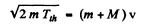(Total mass is constant in the nonrelativistic limit).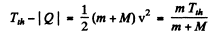or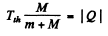Hence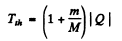6.272. What kinetic energy must a proton possess to split a deuteron H2  whose binding energy is Eb = 2.2 MeV?

Ans. The result of the previous problem applies and we End that energy required to split a deuteron is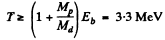6.273. The irradiation of lithium and beryllium targets by a monoergic stream of protons reveals that the reaction Li7(p, n)Be7  -- 1.65 MeV is initiated whereas the reaction Be9(p, n)B9 —  1.85 MeV does not take place. Find the possible values of kinetic energy of the protons.

Ans. Since the reaction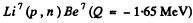is initiated, the incident proton energy must be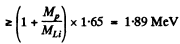since the reaction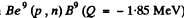is not initiated,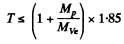= 2.06 MeV Thus 1.89 MeV ≤ T≤ 2.06 MeV

6.274. To activate the reaction (n, a) with stationary B11  nuclei, neutrons must have the threshold kinetic energy Tth = 4.0 MeV. Find the energy of this reaction.

Ans.  We have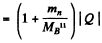or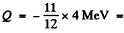-3.67 MeV

6.275. Calculate the threshold kinetic energies of protons required to activate the reactions (p, n) and (p, d) with Li7  nuclei.

Ans. The Q o f the reaction Li7 (p, n ) Be7 was calculated in problem 266 (a). It is - 1.64 MeV Hence, the threshold K.E. of protons for initiating this reaction is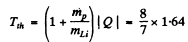For the reaction Li7 (p, d) Li6

we find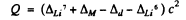= (0.01601 + 0.00783 - 0.01410 - 0.01513) amu x c2

= - 5 02 MeV

The threshold proton energy for initiating this reaction is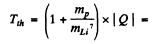5.73 MeV

6.276. Using the tabular values of atomic masses, find the threshold kinetic energy of an alpha particle required to activate the nuclear reaction Li7 (α, n) Be9. What is the velocity of the B10 nucleus in this case?

Ans. The Q of Li7 (a, n) B10 was calculated in problem 266 (c). It is Q = 2.79 MeV Then the threshold energy of a-particle is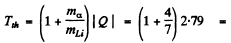4.38MeV

The velocity of B10 in this case is simply the volocity of centre of mass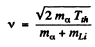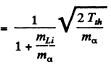This is because both B10 and n are at rest in the CM frame at theshold. Substituting the values of various quantities

we get               v =  5.27 x 106 m/s

6.277. A neutron with kinetic energy T = 10 MeV activates a nuclear reaction C12  (n, α) Be9  whose threshold is Tth = 6.17 MeV. Find the kinetic energy of the alpha-particles outgoing at right angles to the incoming neutrons' direction.

Ans. The momentum of incident neutron is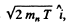that of α particle is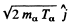and of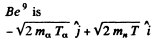By conservation of energy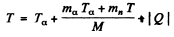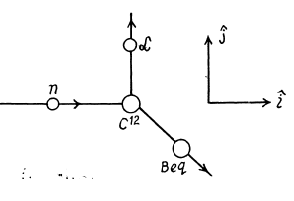(M is the mass of Be9). Thus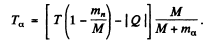Using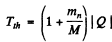we get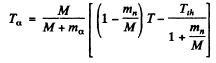M’ is the mass of C12 nucleus.

or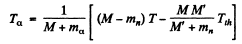= 2.21MeV

6.278. How much, in per cent, does the threshold energy of gamma quantum exceed the binding energy of a deuteron (Eb = 2.2 MeV) in the reaction Y  + H2  → n + p?

Ans.  The formula of problem 6.271 does not apply here because the photon is always reletivistic. At threshold, the energy o f the photon Ey implies a momentum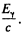The velocity o f centre of mass with respect to the rest frame of initial H2 is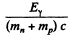Since both n & p are at rest in CM frame at threshold, we write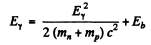by conservation of energy. Since the first term is a small correction, we have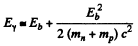Thus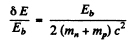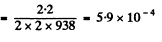or nearly 0.06%

6.279. A proton with kinetic energy T = 1.5 MeV is captured by a deuteron H2. Find the excitation energy of the formed nucleus.

Ans. The reaction is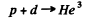Excitation energy of He3 is just the energy available in centre of mass. The velocity of the centre of mass is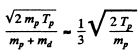In the CM frame, the kinetic energy available is (md ≈ 2 mp)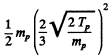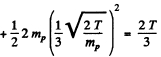The total energy available is then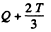where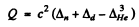= c2 x (0.00783 + 0.01410 - 0.01603) amu

= 5.49 McV

Finally           E = 6.49 MeV.

6.280. The yield of the nuclear reaction C13(d, n)N14 has maximum magnitudes at the following values of kinetic energy Tof bombarding deuterons: 0.60, 0.90, 1.55, and 1.80 MeV. Making use of the table of atomic masses, find the corresponding energy levels of the transitional nucleus through which this reaction proceeds.

Ans. The reaction is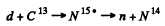Maxima of yields deteimine the eneigy levels of N15 *. As in the previous problem the excitation eneigy is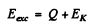where EK = available kinetic eneigy. This is found is as in the previous problem. The velocity of the centre of mass is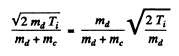so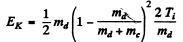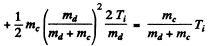Q is the Q value for the ground state of N15 : We have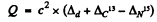= c2 x (0.01410 + 0.00335 - 0.00011) amu

= 16.14 MeV

The excitation energies then are

16.66 MeV, 16.92 MeV

17.49 MeV and 17.70 MeV.

6.281. A narrow beam of thermal neutrons is attenuated η = 360 times after passing through a cadmium plate of thickness d = 0.50 mm. Determine the effective cross-section of interaction of these neutrons with cadmium nuclei.

Ans. We have the relation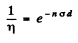Here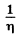attenuation factor

n = no. of Cd nuclei per unit volume

σ = effective cross section

d = thickness of the plate

Now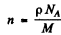(p = density, M = Molar weight of Cd, NA = Avogadro number.)

Thus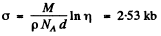6.282. Determine how many times the intensity of a narrow beam of thermal neutrons will decrease after passing through the heavy water layer of thickness d = 5.0 cm. The effective cross-sections of interaction of deuterium and oxygen nuclei with thermal neutrons are equal to σ1 = 7.0 b and σ2  --- 4.2 b respectively.

Ans. Here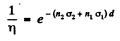where 1 refers to O1 and 2 to D nuclei

Using n2 = 2n, n1 = n = concentration of O nuclei in heavy water we get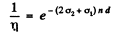Now using the data for heavy water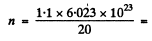3.313 x 1022 per cc

Thus substituting the values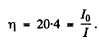6.283. A narrow beam of thermal neutrons passes through a plate of iron whose absorption and scattering effective cross-sections are equal to σa = 2.5b and σ8 = 11b respectively. Find the fraction of neutrons quitting the beam due to scattering if the thickness of the plate is d = 0.50 cm.

Ans. In traversing a distance d the fraction which is either scattered or absorbed is clearly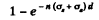by the usual definition of the attenuation factor. Of this, the fraction scattered is (by definition of scattering and absorption cross section)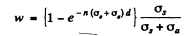In iron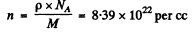Substitution gives                w = 0.352

6.284. The yield of a nuclear reaction producing radionuclides may be described in two ways: either by the ratio w of the number of nuclear reactions to the number of bombarding particles, or by the quantity k, the ratio of the activity of the formed radionuclide to the number of bombarding particles, Find: (a) the half-life of the formed radionuclide, assuming w and k to be known; (b) the yield w of the reaction Li7(p, n)Be7  if after irradiation of a lithium target by a beam of protons (over t = 2.0 hours and with beam current I = 10μA) the activity of Be7 became equal to A = = 1.35.108  dis/s and its half-life to T = 53 days.

Ans. (a) Assuming of course, that each reaction produces a radio nuclide of the same type, the decay constant α of the radionuclide is k/w . Hence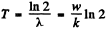(b) number of bombarding particles is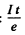(e = charge on proton). Then the number of Be7 produced is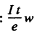If λ = decay constant of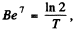, then the activity is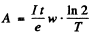Hence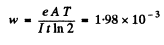6.285. Thermal neutrons fall normally on the surface of a thin gold foil consisting of stable Au197  nuclide. The neutron flux density is J = 1.0.1010  part./(s- cm2). The mass of the foil is m = 10 mg. The neutron capture produces beta-active Au188  nuclei with half-life T = 2.7 days. The effective capture cross-section is σ = 98 b. Find: (a) the irradiation time after which the number of Au187  nuclei decreases by = 1.0%; (b) the maximum number of Au198 nuclei that can be formed during protracted irradiation.

Ans. (a) Suppose - No. of Au197 nuclei in the foil. Then the number of Au197 nuclei transformed in time t is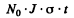For this to equal ηN0, we must have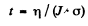= 323 years

(b) Rate of formation of the Au198 nuclei is N0 • J • a per sec
and rate of decay is λn, where n is the number of Au198 at any in stant

Thus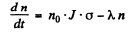The maximum number of Au198 is clearly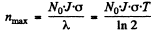because if n is smaller,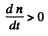and n increase further and if n is larger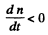and n will decrease. (Actually nmax is approached steadily as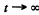)

Substitution give susing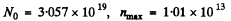6.286. A thin foil of certain stable isotope is irradiated by thermal neutrons falling normally on its surface. Due to the capture of neutrons a radionuclide with decay constant λ appears. Find the law describing accumulation of that radionuclide N (t) per unit area of the foil's surface. The neutron flux density is J, the number of nuclei per unit area of the foil's surface is n, and the effective crosssection of formation of active nuclei is α.

Ans. Rate of formation of the radionuclide is n.J.σ per unit area per-sec. Rate of decay is λN.
Thus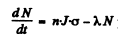per unit area per second

Then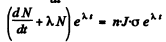or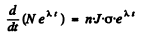Hence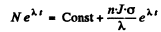The number of radionuclide at t = 0 when the process starts is zero. So constant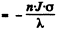Then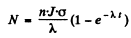6.287. A gold foil of mass m = 0.20g was irradiated during t = 6.0 hours by a thermal neutron flux falling normally on its surface. Following ζ = 12 hours after the completion of irradiation the activity of the foil became equal to A = 1.9.107 dis/s. Find the neutron flux density if the effective cross-section of formation of a radioactive nucleus is σ = 96b, and the half-life is equal to T = 2.7 days.

Ans. We apply the formula of the previous problem except that have N = no. of radio nuclide and no. of host nuclei originally.

Here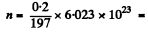6.115 x1020

Then after time t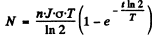T = half life of the radionuclide.

After the source of neutrons is cut off the activity after time T will be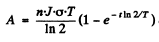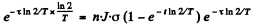Thus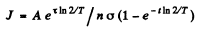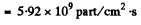6.288. How many neutrons are there in the hundredth generation if the fission process starts with N = 1000 neutrons and takes place in a medium with multiplication constant k = 1.05?

Ans. No. of nuclei in the first generation = No .of nuclei initially = N0

N0 in the second generation = Nx multiplication factor = N0.k

N0 in the the 3rd generation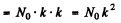N0 in the nth generationSubstitution gives 1.25 x 105 neutrons

6.289. Find the number of neutrons generated per unit time in a uranium reactor whose thermal power is P = 100 MW if the average number of neutrons liberated in each nuclear splitting is v = 2.5. Each splitting is assumed to release an energy E = = 200 MeV.

Ans. No- of fissions per unit time is clearly P/E. Hence no. of neutrons produced per unit time to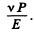Substitution gives 7.80 x 1018 neutrons/sec

6.290. In a thermal reactor the mean lifetime of one generation of thermal neutrons is ζ = 0.10 s. Assuming the multiplication constant to be equal to k = 1.010, find: (a) how many times the number of neutrons in the reactor, and consequently its power, will increase over t = 1.0 min;
(b) the period T of the reactor, i.e. the time period over which its power increases e-fold.

Ans. (a) This number is kn - 1 where n = no. of generations in time t = t/T

Substitution gives 388.

(b) We write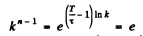or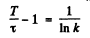andThe document Irodov Solutions: Nuclear Reactions- 2 | I. E. Irodov Solutions for Physics Class 11 & Class 12 - JEE is a part of the JEE Course I. E. Irodov Solutions for Physics Class 11 & Class 12.
All you need of JEE at this link: JEE

106 docs

## FAQs on Irodov Solutions: Nuclear Reactions- 2 - I. E. Irodov Solutions for Physics Class 11 & Class 12 - JEE

 1. What is a nuclear reaction?Ans. A nuclear reaction refers to a process in which the nucleus of an atom undergoes a change, resulting in the formation of different isotopes or elements. These reactions involve the splitting of atomic nuclei (nuclear fission) or the combining of atomic nuclei (nuclear fusion).
 2. What are the different types of nuclear reactions?Ans. There are two main types of nuclear reactions: nuclear fission and nuclear fusion. In nuclear fission, the nucleus of an atom is split into two or more smaller nuclei, releasing a large amount of energy. Nuclear fusion, on the other hand, involves the combining of two or more atomic nuclei to form a larger nucleus, also releasing a substantial amount of energy.
 3. How does a nuclear reaction produce energy?Ans. Nuclear reactions produce energy through a process called nuclear binding energy. During a nuclear reaction, the total mass of the reactants is slightly larger than the mass of the products. This difference in mass is converted into energy according to Einstein's mass-energy equivalence principle (E = mc^2), where E is energy, m is the mass difference, and c is the speed of light.
 4. What are the applications of nuclear reactions?Ans. Nuclear reactions have various applications, including the generation of electricity in nuclear power plants, the production of medical isotopes for diagnostic and therapeutic purposes, and the propulsion of nuclear-powered submarines and spacecraft. Nuclear reactions also play a crucial role in nuclear weapons.
 5. How do nuclear reactions contribute to radioactive decay?Ans. Nuclear reactions can lead to the formation of radioactive isotopes, which subsequently undergo radioactive decay. During radioactive decay, unstable isotopes emit radiation in the form of alpha particles, beta particles, or gamma rays to achieve a more stable state. This decay process continues until a stable isotope is reached.

## I. E. Irodov Solutions for Physics Class 11 & Class 12

106 docsExplore Courses for JEE examSignup to see your scores go up within 7 days! Learn & Practice with 1000+ FREE Notes, Videos & Tests.
10M+ students study on EduRev
Track your progress, build streaks, highlight & save important lessons and more!
Related Searches

,

,

,

,

,

,

,

,

,

,

,

,

,

,

,

,

,

,

,

,

,

;